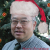## c++ 虚函数的语法知识

【福利】CTO直接拍板说好用的SDK全在这里了！0元配齐，全部免费！>>>virtual void set() =0;
virtual void show() const =0;

01. 在许多情况下，在基类中不能对虚函数给出有意义的实现，而把它声明为纯虚函数，它的实现留给该基类的派生类去做。这就是纯虚函数的作用。楼主的两行代码声明的函数，均为 纯虚函数。
2. const用在成员函数后的目的，主要是针对类的 const 对象。就是说：const对象只能调用const成员函数；const对象的值不能被修改，即在const成员函数中修改const对象数据成员的值是语法错误；在const函数中调用非const成员函数也是语法错误。

``````#include <iostream>
using namespace std;

class   R_Base{
public:
virtual  void   show() = 0;
virtual  void   show() const = 0;

};

class R: public R_Base {
public:
R(int   r1,   int   r2)   {   R1=r1;   R2=r2;   }
void  show()   {
cout<<R1<<", "<<R2<<" 调用为非常量对象所用的 show()。"<<endl;
}

void  show()const{
cout<<R1<<", "<<R2<<" 调用为常量对象所用的 show()。"<<endl;
}

private :
int   R1,   R2;
};

int   main(){
R   a(5,   4);
a.show();   // 调用不带 const 的函数
const   R   b(20,   52);
b.show();   // 常量对象只能调用带 const 的函数

return 0;
}``````

``````5, 4 调用为非常量对象所用的 show()。
20, 52 调用为常量对象所用的 show()。``````